# Data Handling Class 7 Notes: Chapter 3

## Double Bar Graphs

Double bar graphs are an effective tool to compare the values of two quantities for the same observation. For example, consider the marks obtained by five students of a class in two tests. Using a double bar graph, we can analyse which week students had better marks.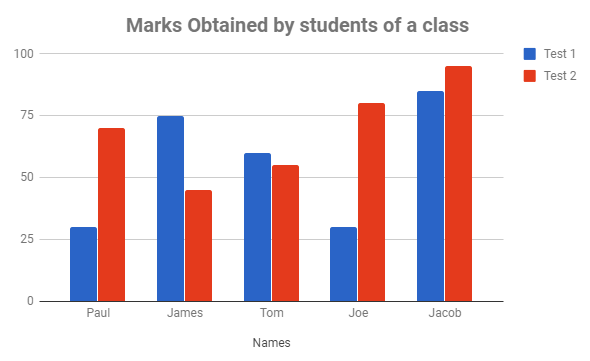#### For more information on Double Bar Graphs, watch the below video.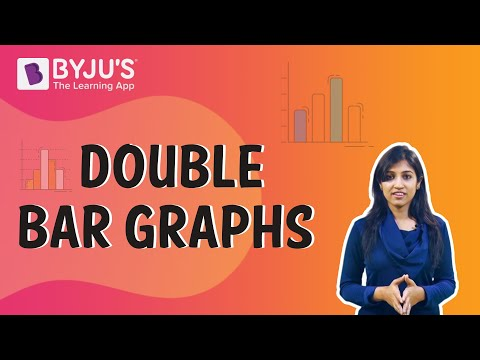To know more about Double Bar Graphs, visit here.

## Averages

### Arithmetic Mean and Range

The average or arithmetic mean or mean of a given data is defined as :

Mean=Sum of all observations / Number of observations

The difference between the highest and the lowest observations in a given data is called its Range.
Example: Ages of all 10 teachers in grade 7 are : 25, 43, 34, 55, 44, 60, 32, 29, 35, 40.
Mean = 43+34+55+44+60,+32+29+35+40 = 39.7 years
Range = Higest Observation – Lowest Observation = 60 – 25 = 35

To know more about Averages, visit here.

### Median

When a given data is arranged in ascending (or descending) order, then the middlemost observation is called the median of the data.
Example : Marks scored by seven students in a class are: 21, 32, 18 ,93, 21, 36, 50.
Observations in ascending order: 18, 21, 21, 32, 36, 50, 93.
Middle most value = 32
∴ Median is 32.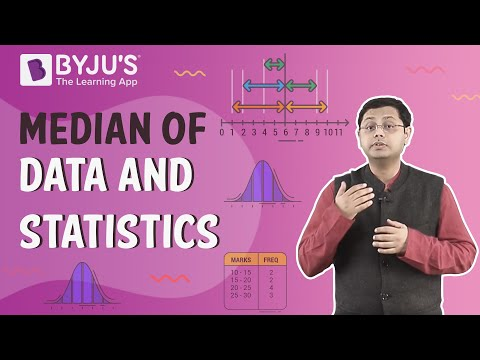To know more about Median, visit here.

### Mode

The mode of a set of observations is the observation that occurs most often.
Example: Given set of numbers: 1, 1, 2, 4, 3, 2, 1, 2, 2, 4
Ascending Order = 1, 1, 1, 2, 2, 2, 2, 3, 4, 4
∴ Mode of this data is 2 because it occurs more frequently.
Note: A data can have more than 1 mode.

To know more about Mode, visit here.

## Chance and Probability

Probability is the measure or the chance of occurrence of a particular event. Experiments which do not have a fixed result are known as random experiments.

Number of outcomes or Sample Space  The set of all the possible outcomes to occur in any experiment is known as sample space.
Examples: Experiment : Tossing a coin, Sample Space (S) = {H,T}

Experiment : Rolling a die, Sample Space (S) = {1,2,3,4,5,6}
Favourable outcome – It is one of the possible result(s) of an experiment.
Examples : In an experiment of Tossing a coin, getting a head. Favourable outcome = {H} In an experiment of Rolling a die, getting an even number Favourable outcomes = {2,4,6} Probability of occurrence of any event,

$$\begin{array}{l}P(E)=\frac{Number\,of\,favourable\,outcomes}{Total\,Number\,of\,Outcomes}\end{array}$$

Example: Find the probability of getting an even number when a die is rolled. Sample Space (S) = {1,2,3,4,5,6} , Favourable outcomes = {2,4,6}

$$\begin{array}{l}P(E)=\frac{Number\,of\,favourable\,outcomes}{Total\,Number\,of\,Outcomes}=\frac{3}{6}=\frac{1}{2}\end{array}$$

#### For more information on Chance and Probability, watch the below video.To know more about Chance and Probability, visit here.

## The Scale

Large numbers cannot be represented in a bar graph, so the scaling factor is used to reduce or scale down large numbers.

Example: The scale on the y-axis is 1 unit = 200 people.

## Basics: The Right Data in the Right Form

### Introduction: Data

Data are individual pieces of information, information about a particular system. They can be in the form of figures or numbers. Data is collected to analyse specific information for a specific purpose.

To know more about Data, visit here.

### Organization of Data

Data is organised and represented graphically so that it becomes easy to understand and interpret. This is called an organisation of data.

#### For more information on Organisation of Data, watch the below video.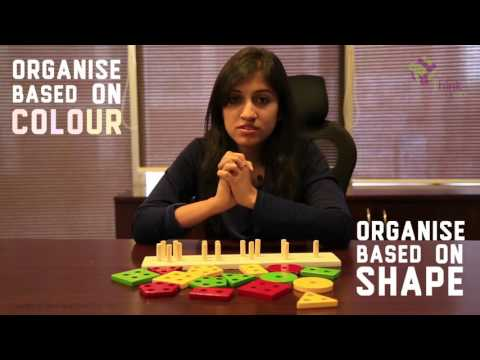To know more about Data Organisation, visit here.

## Pictographs and Bar Graphs

A pictograph is a pictorial representation of data. Here data is represented using images of the objects.

The graphical representation of data using bars of uniform width drawn vertically or horizontally with different lengths is called as bar graphs/bar diagrams. Bar diagrams consist of two axes: X-axis and Y-axis. The following is a bar graph showing the birthday of students in a class. Graph showing the birthday of students in a class.

#### For more information on Pictographs and Bar Graphs, watch the below videos.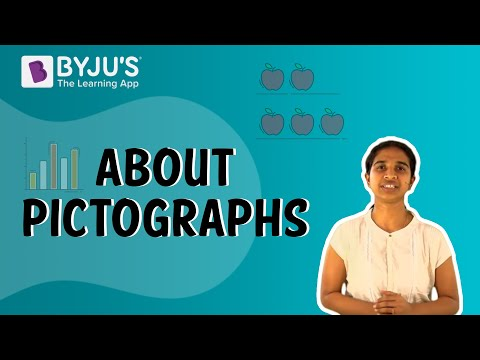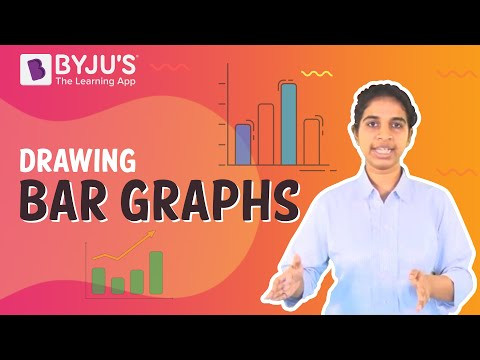## Frequently asked Questions on CBSE Class 7 Maths Notes Chapter 3 Data Handling

Q1

### What is a double bar graph?

A double bar graph is used to display two sets of data on the same graph.

Q2

### What is a scaling factor?

A scale factor is a number which multiplies (“scales”) a quantity

Q3

### What is the meaning of ‘Favourable outcome’?

A favourable outcome is an outcome that denotes how many times we expect the thing to happen.

To know more about Bar Graph, visit here.